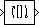# Flip

Flip input vertically or horizontally

## Library

Signal Management / Indexing

`dspindex`

•## Description

The Flip block vertically or horizontally reverses the M-by-N input matrix, u. The output always has the same dimensionality as the input.

When you set the Flip along parameter to `Columns`, the block flips the input vertically so the first row of the input becomes the last row of the output.

```y = flipud(u) % Equivalent MATLAB code ```

When flipping the input vertically, the block treats length-M unoriented vector inputs as M-by-1 column vectors.

When you set the Flip along parameter to `Rows`, the block flips the input horizontally so the first column of the input becomes the last column of the output.

```y = fliplr(u) % Equivalent MATLAB code ```

When flipping the input horizontally, the block treats length-N unoriented vector inputs as 1-by-N row vectors.

This block supports Simulink® virtual buses.

## Parameters

Flip along

Specify the dimension along which to flip the input. When you set this parameter to `Columns`, the block flips the input vertically. When you set this parameter to `Rows`, the block flips the input horizontally.

## Supported Data Types

PortSupported Data Types

Input

• Double-precision floating point

• Single-precision floating point

• Fixed point (signed and unsigned)

• Boolean

• 8-, 16-, and 32-bit signed integers

• 8-, 16-, and 32-bit unsigned integers

• Enumerated

Output

• Double-precision floating point

• Single-precision floating point

• Fixed point (signed and unsigned)

• Boolean

• 8-, 16-, and 32-bit signed integers

• 8-, 16-, and 32-bit unsigned integers

• Enumerated

 Selector (Simulink) Simulink Variable Selector DSP System Toolbox `flipud` MATLAB `fliplr` MATLAB